# Bezier Curve in Computer Graphics | Examples

## Bezier Curve-

Bezier Curve may be defined as-

• Bezier Curve is parametric curve defined by a set of control points.
• Two points are ends of the curve.
• Other points determine the shape of the curve.

The concept of bezier curves was given by Pierre Bezier.

## Bezier Curve Example-

The following curve is an example of a bezier curve-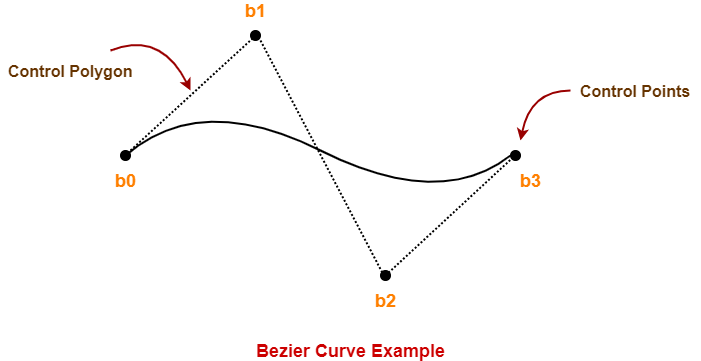Here,

• This bezier curve is defined by a set of control points b0, b1, b2 and b3.
• Points b0 and b3 are ends of the curve.
• Points b1 and b2 determine the shape of the curve.

## Bezier Curve Properties-

Few important properties of a bezier curve are-

### Property-01:

Bezier curve is always contained within a polygon called as convex hull of its control points.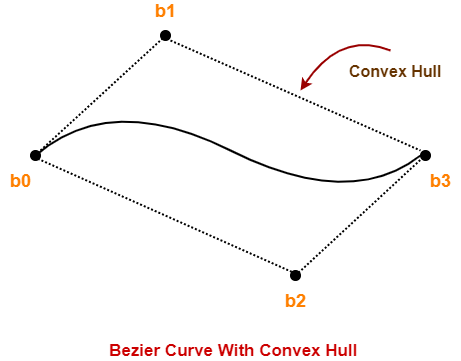### Property-02:

• Bezier curve generally follows the shape of its defining polygon.
• The first and last points of the curve are coincident with the first and last points of the defining polygon.

### Property-03:

The degree of the polynomial defining the curve segment is one less than the total number of control points.

Degree = Number of Control Points – 1

### Property-04:

The order of the polynomial defining the curve segment is equal to the total number of control points.

Order = Number of Control Points

### Property-05:

• Bezier curve exhibits the variation diminishing property.
• It means the curve do not oscillate about any straight line more often than the defining polygon.

## Bezier Curve Equation-

A bezier curve is parametrically represented by-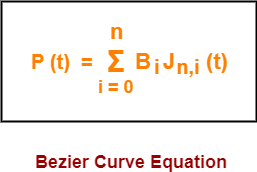Here,

• t is any parameter where 0 <= t <= 1
• P(t) = Any point lying on the bezier curve
• Bi = ith control point of the bezier curve
• n = degree of the curve
• Jn,i(t) = Blending function = C(n,i)ti(1-t)n-i where C(n,i) = n! / i!(n-i)!

## Cubic Bezier Curve-

• Cubic bezier curve is a bezier curve with degree 3.
• The total number of control points in a cubic bezier curve is 4.

### Example-

The following curve is an example of a cubic bezier curve-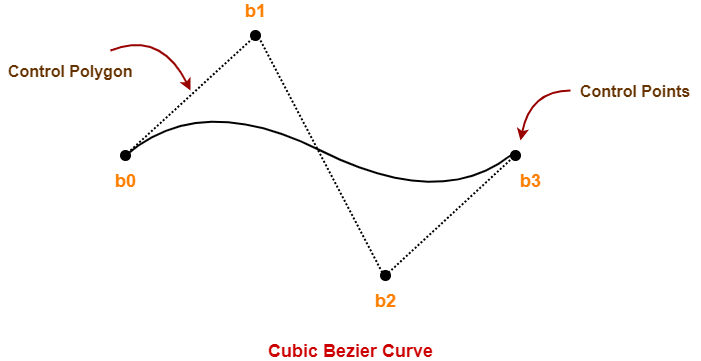Here,

• This curve is defined by 4 control points b0, b1, b2 and b3.
• The degree of this curve is 3.
• So, it is a cubic bezier curve.

### Cubic Bezier Curve Equation-

The parametric equation of a bezier curve is-Substituting n = 3 for a cubic bezier curve, we get-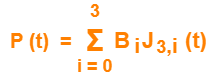Expanding the above equation, we get-

P (t) = B0J3,0(t) + B1J3,1(t) + B2J3,2(t) + B3J3,3(t)     ………..(1)

Now,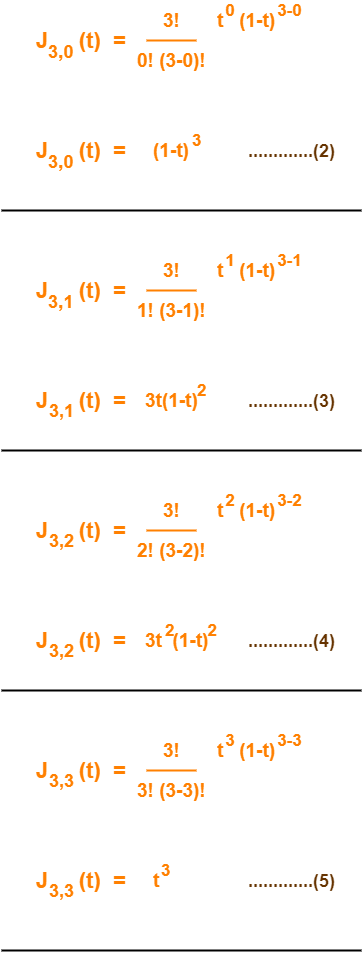Using (2), (3), (4) and (5) in (1), we get-

P(t) = B0(1-t)3 + B13t(1-t)2 + B23t2(1-t) + B3t3

This is the required parametric equation for a cubic bezier curve.

## Applications of Bezier Curves-

Bezier curves have their applications in the following fields-

### 1. Computer Graphics-

• Bezier curves are widely used in computer graphics to model smooth curves.
• The curve is completely contained in the convex hull of its control points.
• So, the points can be graphically displayed & used to manipulate the curve intuitively.

### 2. Animation-

• Bezier curves are used to outline movement in animation applications such as Adobe Flash and synfig.
• Users outline the wanted path in bezier curves.
• The application creates the needed frames for the object to move along the path.
• For 3D animation, bezier curves are often used to define 3D paths as well as 2D curves.

### 3. Fonts-

• True type fonts use composite bezier curves composed of quadratic bezier curves.
• Modern imaging systems like postscript, asymptote etc use composite bezier curves composed of cubic bezier curves for drawing curved shapes.

## Problem-01:

Given a bezier curve with 4 control points-

B0[1 0] , B1[3 3] , B2[6 3] , B3[8 1]

Determine any 5 points lying on the curve. Also, draw a rough sketch of the curve.

## Solution-

We have-

• The given curve is defined by 4 control points.
• So, the given curve is a cubic bezier curve.

The parametric equation for a cubic bezier curve is-

P(t) = B0(1-t)3 + B13t(1-t)2 + B23t2(1-t) + B3t3

Substituting the control points B0, B1, B2 and B3, we get-

P(t) = [1 0](1-t)3 + [3 3]3t(1-t)2 + [6 3]3t2(1-t) + [8 1]t3      ……..(1)

Now,

To get 5 points lying on the curve, assume any 5 values of t lying in the range 0 <= t <= 1.

Let 5 values of t are 0, 0.2, 0.5, 0.7, 1

### For t = 0:

Substituting t=0 in (1), we get-

P(0) = [1 0](1-0)3 + [3 3]3(0)(1-t)2 + [6 3]3(0)2(1-0) + [8 1](0)3

P(0) = [1 0] + 0 + 0 + 0

P(0) = [1 0]

### For t = 0.2:

Substituting t=0.2 in (1), we get-

P(0.2) = [1 0](1-0.2)3 + [3 3]3(0.2)(1-0.2)2 + [6 3]3(0.2)2(1-0.2) + [8 1](0.2)3

P(0.2) = [1 0](0.8)3 + [3 3]3(0.2)(0.8)2 + [6 3]3(0.2)2(0.8) + [8 1](0.2)3

P(0.2) = [1 0] x 0.512 + [3 3] x 3 x 0.2 x 0.64 + [6 3] x 3 x 0.04 x 0.8 + [8 1] x 0.008

P(0.2) = [1 0] x 0.512 + [3 3] x 0.384 + [6 3] x 0.096 + [8 1] x 0.008

P(0.2) = [0.512 0] + [1.152 1.152] + [0.576 0.288] + [0.064 0.008]

P(0.2) = [2.304 1.448]

### For t = 0.5:

Substituting t=0.5 in (1), we get-

P(0.5) = [1 0](1-0.5)3 + [3 3]3(0.5)(1-0.5)2 + [6 3]3(0.5)2(1-0.5) + [8 1](0.5)3

P(0.5) = [1 0](0.5)3 + [3 3]3(0.5)(0.5)2 + [6 3]3(0.5)2(0.5) + [8 1](0.5)3

P(0.5) = [1 0] x 0.125 + [3 3] x 3 x 0.5 x 0.25 + [6 3] x 3 x 0.25 x 0.5 + [8 1] x 0.125

P(0.5) = [1 0] x 0.125 + [3 3] x 0.375 + [6 3] x 0.375 + [8 1] x 0.125

P(0.5) = [0.125 0] + [1.125 1.125] + [2.25 1.125] + [1 0.125]

P(0.5) = [4.5 2.375]

### For t = 0.7:

Substituting t=0.7 in (1), we get-

P(t) = [1 0](1-t)3 + [3 3]3t(1-t)2 + [6 3]3t2(1-t) + [8 1]t3

P(0.7) = [1 0](1-0.7)3 + [3 3]3(0.7)(1-0.7)2 + [6 3]3(0.7)2(1-0.7) + [8 1](0.7)3

P(0.7) = [1 0](0.3)3 + [3 3]3(0.7)(0.3)2 + [6 3]3(0.7)2(0.3) + [8 1](0.7)3

P(0.7) = [1 0] x 0.027 + [3 3] x 3 x 0.7 x 0.09 + [6 3] x 3 x 0.49 x 0.3 + [8 1] x 0.343

P(0.7) = [1 0] x 0.027 + [3 3] x 0.189 + [6 3] x 0.441 + [8 1] x 0.343

P(0.7) = [0.027 0] + [0.567 0.567] + [2.646 1.323] + [2.744 0.343]

P(0.7) = [5.984 2.233]

### For t = 1:

Substituting t=1 in (1), we get-

P(1) = [1 0](1-1)3 + [3 3]3(1)(1-1)2 + [6 3]3(1)2(1-1) + [8 1](1)3

P(1) = [1 0] x 0 + [3 3] x 3 x 1 x 0 + [6 3] x 3 x 1 x 0 + [8 1] x 1

P(1) = 0 + 0 + 0 + [8 1]

P(1) = [8 1]

Following is the required rough sketch of the curve-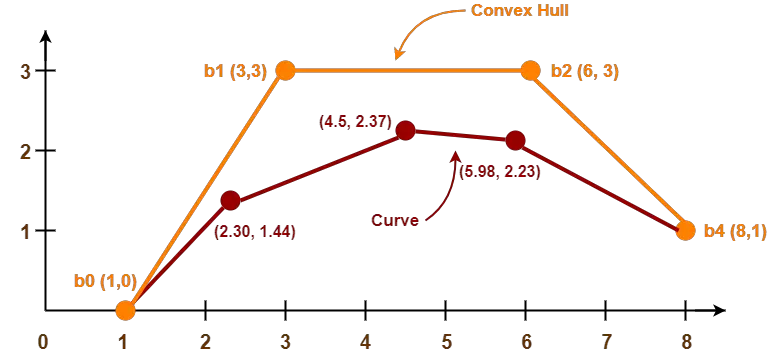To gain better understanding about Bezier Curves in Computer Graphics,

Watch this Video Lecture

Get more notes and other study material of Computer Graphics.

Watch video lectures by visiting our YouTube channel LearnVidFun.

SummaryArticle Name
Bezier Curve in Computer Graphics | Examples
Description
Bezier Curve in Computer Graphics is a parametric curve defined by a set of control points. Bezier Curve Example & Properties. Cubic Bezier Curve is a bezier curve with degree 3. Problem on Bezier Curve.
Author
Publisher Name
Gate Vidyalay
Publisher Logo